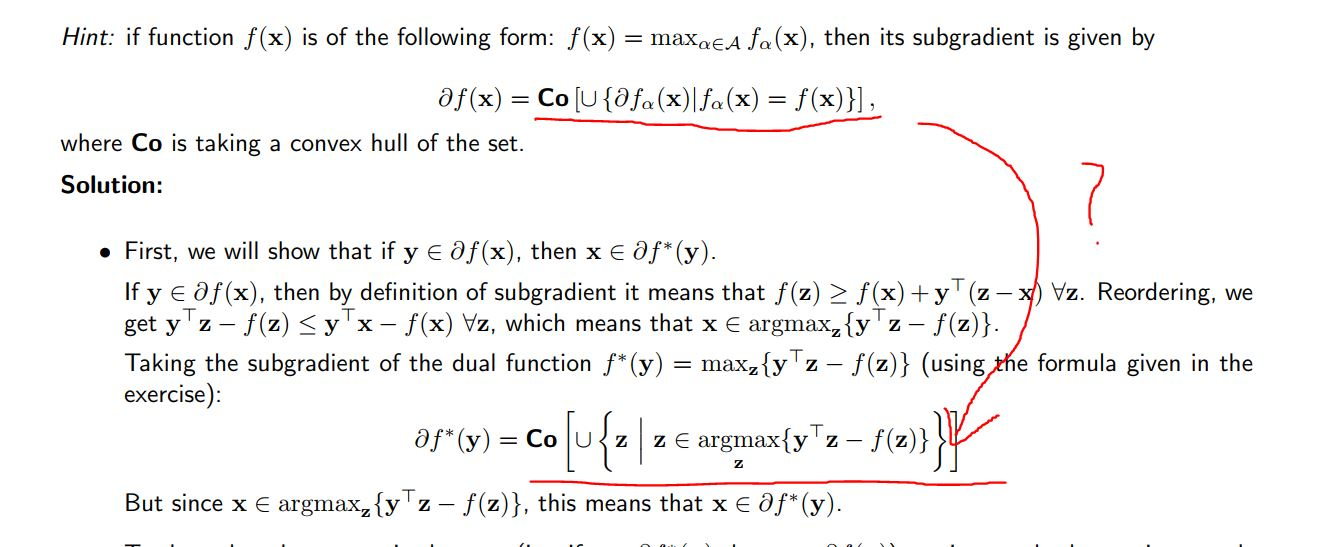### Question for exercise 10

Hi,

I have a question about exercise 10.
We are given an expression for the subgradient of a max function as the hint.
And in the solution, after applying the hint, the subgradients is a set of argmax of the dual function. I do not get it. Could you please elaborate a little bit more? Thank you.Top comment

Hello,

Good question. There are two key things to observe here:

1. $$\text{argmax}_z y^\top z - f(z)$$ means "$$z$$ for which the function is maximized". That's a very similar selection criterion as above.
2. $$z$$ is the gradient of $$y\top z - f(z)$$ (w.r.t. $$y$$)

Hope this helps!

Page 1 of 1